### Vol 17, No 10 (2017) / Huang

Scattering Law Analysis Based on Hapke and Lommel-Seeliger Models for Asteroidal Taxonomy

# Scattering Law Analysis Based on Hapke and Lommel-Seeliger Models for Asteroidal Taxonomy

Huang Xiang-Jie1, Lu Xiao-Ping1, 2, 4, *, Li Jian-Yang5, Mei Bao1, 2, Hsia Chih-Hao1, 2, Zhao Hai-Bin3, 4

Macau University of Science and Technology, Taipa, Macau, China
Lunar and Planetary Science Laboratory, Macau University of Science and Technology - Partner Laboratory of Key Laboratory of Lunar and Deep Space Exploration, Chinese Academy of Sciences, Taipa 999078, Macau, China
Purple Mountain Observatory, Chinese Academy of Sciences, Nanjing 210008, China
Key Laboratory of Planetary Sciences, Chinese Academy of Sciences, Nanjing 210008, China
Planetary Science Institute, 1700 E. Ft. Lowell Road, Suite 106, Tucson, AZ 85719, USA

† Corresponding author. E-mail: xplu@must.edu.mo

Abstract: Abstract

In deriving the physical properties of asteroids from their photometric data, the scattering law plays an important role, although the shape variations of asteroids result in the main variations in lightcurves. By following the physical behaviors of light reflections, Hapke et al. deduced complex functions to represent the scattering process, however, it is very hard to accurately simulate the surface scattering law in reality. For simplicity, other numerical scattering models are presented for efficiently calculating the physical properties of asteroids, such as the Lommel-Seeliger (LS) model. In this article, these two models are compared numerically. It is found that in some numerical applications the LS model in simple form with four parameters can be exploited to replace the Hapke model in complex form with five parameters. Furthermore, the generated synthetic lightcurves by the Cellinoid shape model also show that the LS model can perform as well as the Hapke model in the inversion process. Finally, by applying the Principal Component Analysis (PCA) technique to the parameters of the LS model, we present an efficient method to classify C and S type asteroids, instead of the conventional method using the parameters of the Hapke model.

Keywords: scattering;techniques: photometric;minor planets;asteroids: general

## 1. Introduction

Being composed of primitive matter from the early solar system, an asteroid can reveal the evolution mechanism of planets and provide information on the formation of our solar system (DeMeo & Carry ). By using ground-based observations, the physical properties of asteroids can be derived from their photometric data such as rotational periods, pole orientations and overall shapes (Lu et al. , ; Lu & Ip ; Lu et al. ).

In deriving the physical properties of asteroids from their photometry data, the scattering law plays an important role, although the shape variations of asteroids result in the main variations in their lightcurves (Karttunen ; Karttunen & Bowell ). As shown in Figure 1, the scattering geometry can be defined in terms of the angle of incidence i between the light source and local surface normal, the angle of emergence e between the observer and the local surface normal, and the phase angle α between the light source and observer, as seen from the object, in addition to the azimuthal angle ϕ between the planes of incidence and emergence. By following the physical behaviors of light reflections, Hapke (, , ) and Hapke & Wells () deduced complex functions to represent the scattering process. Their evolved model has been applied extensively to spacecraft and telescopic observations for a lot of objects throughout the solar system to derive their physical and surface characteristics (Li et al. , , , , ).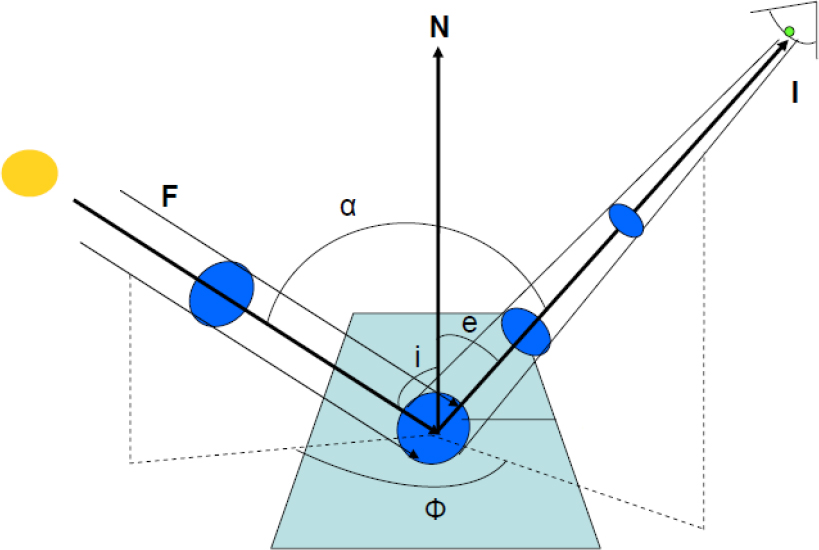Fig. 1 Schematic diagram illustrating bidirectional reflectance with solar phase angle (α), angle of incidence (i) and angle of emergence (e).

However, application of the Hapke model is very complicated when applying a numerical inversion process to investigate the physical properties of asteroids. There are many equivalent methods that have attempted to numerically replace it such as the joint linear exponential model published by Muinonen et al. (), the Minnaert model proposed by Minnaert (), and the Lommel-Seeliger (LS) model constructed by Seeliger (). In particular, the LS model is very efficient in numerical calculations of synthetic brightnesses of asteroids. Recently,many applications have been developed to derive the physical parameters for asteroids with the LS model (Cellino et al. ; Muinonen & Lumme ).

Parameters in the Hapke model have corresponding physical meanings such as albedo, which is a measure of the amount of reflected light from the surface, and others as described in Table 1. Moreover, the LS model can be applied efficiently to calculate the physical parameters for asteroids (Muinonen et al. ; Wang et al. ). In this article, we make an attempt to combine two models, by presenting an equivalent method to connect the physical meanings of the Hapke model to the parameters of the LS model. Following the method, the parameters of the LS model can be efficiently derived in fitting the photometric data and subsequently they can be analyzed by the Principal Component Analysis (PCA) technique to obtain a classification for asteroids (Abdi & Williams ). Ultimately, to better identify asteroid types, the least absolute shrinkage and selection operator (LASSO) technique is introduced to efficiently classify C and S type asteroids based on LS model parameters (Tibshirani ; Mei & Ling ; Musoro et al. ).

Parameter Symbol Meaning
Single scattering albedo ω Fraction of total incident energy that is scattered by a single particle towards all directions
Asymmetry factor g Spatial energy distribution in a single particle scattering phase function
Opposition surge amplitude B so Amplitude of opposition effect (SHOE only)
Opposition surge width h s Width of opposition effect (SHOE only)
Roughness parameter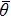Average deviation from local normal with respect to average

Table 1.Physical Meanings of Five Parameters in the Hapke Model.

The paper will be arranged as follows. First of all, in Section 2, the Hapke model and LS model will be concisely presented. In Section 3, numerical simulations are conducted to confirmthat the LS model can performwell as a replacement for the Hapkemodel in the inversion application. Furthermore, equivalent to the Hapke model, the parameters of LS are analyzed by the PCA method for classifying C and S type asteroids. Subsequently, the LASSOmethod is employed for the taxonomy of C and S type asteroids from the LS model parameters. In the end, we sum up the primary work of this article and discuss future work in Section 4.

## 2. Scattering Models

2.1. Hapke Model

The Hapke model is based on a semi-physical model that uses the analytical solutions of radiative transfer on an asteroidal surface with simple assumptions, coupled with empirical models that describe the scattering behavior of the particulate surface (Hapke ).

There are at least five parameters in the Hapke model, including the single scattering albedo (ω), photometric roughness (), amplitude and width of the opposition effect (B so and h s respectively for the shadow-hiding opposition effect (SHOE), and B co and h c respectively for the coherent backscattering opposition effect (CBOE)), as well as one or more parameters to describe the single-particle phase function. The model exploited throughout this article is a five-parameter version, which only considers the SHOE, and adopts a one-term Henyey-Greenstein (HG) phase function (Buratti & Veverka ; Li et al. ) involving one asymmetry parameter g to describe the single-particle phase function. The five parameters are summarized in Table 1 and the radiance factor (RADF), the ratio of bidirectional reflectance of a surface to that of a perfectly diffuse surface illuminated at i = 0° (Hapke ), is shown in Equation (1), where B(α) is the shadow hiding opposition surge function, p(α) is the average particle single-scattering phase function, H(x) is the Ambartsumian-Chandrasekhar function for multiple scattering, and S is the Hapke roughness shadowing function (Hapke ).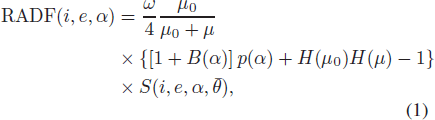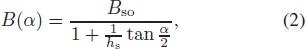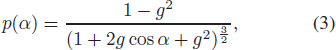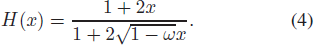2.2. LS Model

The LS model is a widely-used scattering model (Besse et al. ), developed by Seeliger () and improved by Hapke (). The RADF (Takir et al. ) of the LS model with four free parameters is shown in Equation (5), where f(α) = e βα + γα 2 + δα 3 with three polynomial coefficients β, γ and δ that describe the variation in surface reflectance with respect to phase angle α (in degrees) and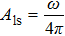is the LS albedo with the average particle single scattering albedo, ω.

Here in both Equation (1) and Equation (2), μ 0 and μ are defined as the projections of the illuminating direction and emitting direction on the normal direction of the surface, respectively.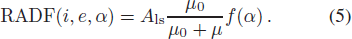## 3. Scattering Law Analysis for Hapke and LS models

Both the Hapke and LS models are explained in the previous section. Intuitively, the LS model has a much simpler form than the Hapke model. In this section, first, the equivalent parameters of the LS model are numerically fitted to the given parameters of the Hapke model. By applying the two equivalent parameters to generate synthetic lightcurves based on a Cellinoid shape model, their similar morphologies of synthetic lightcurves confirm that the LS model can provide the same result as the Hapke model. Then the PCA technique is applied to analyze equivalent parameters of the LS model, derived from the Hapke parameters of C and S type asteroids. Then the result shows that different types of asteroids can be identified from parameters of the LS model.

3.1. Equivalent Parameters

To better simulate the real circumstances, the derived parameters of different scattering models for (101955) Bennu by Takir et al. () are exploited for comparison of the equivalent parameters.

The phase curves of (101955) Bennu for the Hapke model with ω = 0.031, B so = 3.9, h s = 0.11, g = −0.32 and= 20° and the fitted LS model are shown in Figure 2. From Figure 2, it is clear that the LS model can fit the Hapke model very well and the opposition effect in the phase curve is also fitted well. The fitted value of the Sum of Squared Errors (SSE), defined as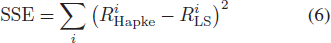is 0.0001312, where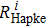and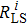respectively denote the reflectances of the Hapke and LS models for the corresponding phase angle i.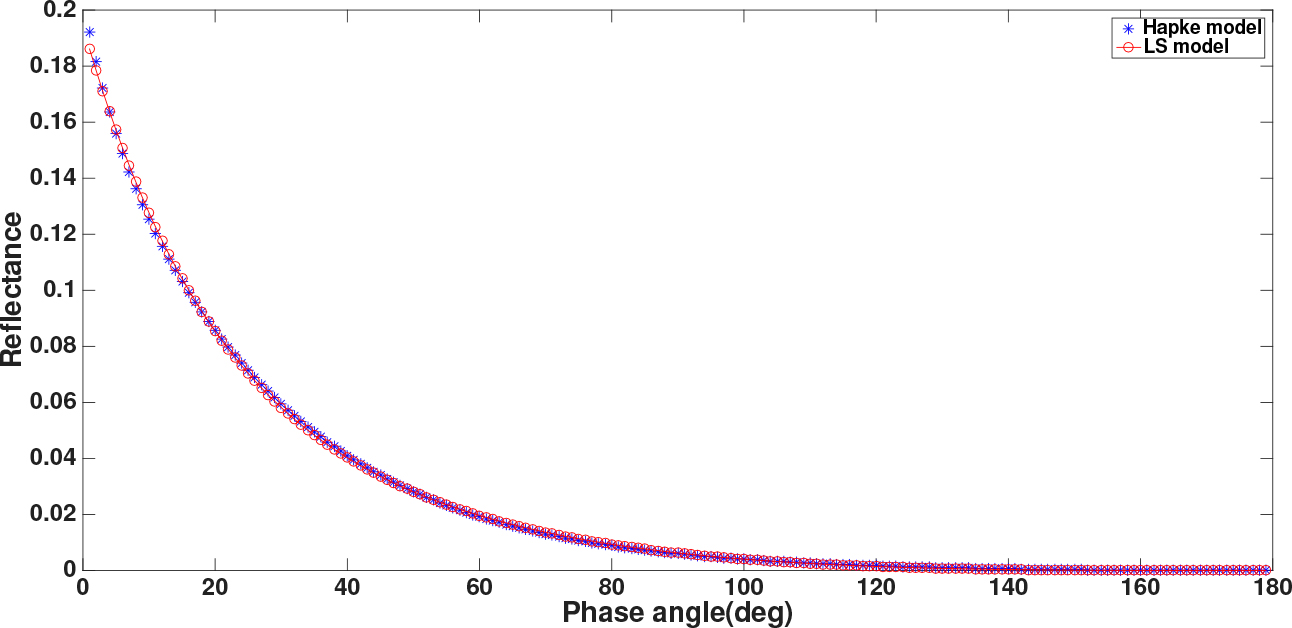Fig. 2 Phase curves of (101955) Bennu for the Hapke (shown as blue ‘∗’) and LS (shown as red ‘◦’) models.

Additionally, the fitted equivalent parameters of the LS model are listed in Table 2. Meanwhile, the parameters of the LS model derived by Takir et al. () are also listed for comparison and confirmation.

Our study Takir et al. ()
A ls 0.0286 0.030
β −4.20 × 10−2 −4.36 × 10−2
γ 2.63 × 10−4 2.69 × 10−4
δ −1.09 × 10−6 −9.90 × 10−7

Table 2 Equivalent Parameters from the LS Model

Furthermore, the parameters of the Hapke model and the fitted equivalent parameters of the LS model listed in Table 2 are applied to generate synthetic lightcurves based on a Cellinoid shape model presented by Lu et al. (), which are shown in Figure 3. Both of the synthetic lightcurves have a similar morphology with the same positions of maximum and minimum. Although deviations between the maximum and minimum of the two synthetic lightcurves exist, the equivalent parameters of the LS model can fit the Hapke model well as the relative brightness is commonly applied in real applications. The slightly larger amplitude of the LS model than the Hapke model will not affect results of the inverse process (Lu et al. ).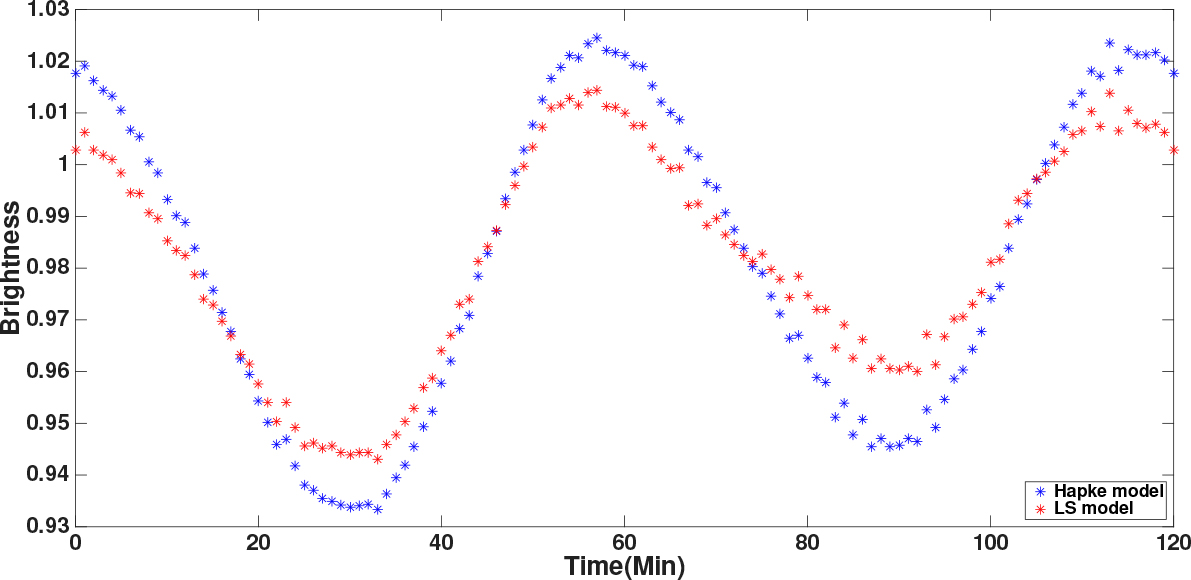Fig. 3 Synthetic lightcurves based on a Cellinoid shape with scattering parameters of the Hapke (blue ‘∗’) and LS (red ‘∗’)models.
3.2. Asteroidal Taxonomy Based on the LS Model

As the parameters in the Hapke model can reveal information about the surface of asteroids, Helfenstein & Veverka () introduced the taxonomy of C and S type asteroids according to the five parameters. The mean parameters are listed in Table 3, where the equivalent parameters of the LS model are also listed. The corresponding phase curves for C and S type asteroids with these mean parameters of Hapke and LS models are shown in Figure 4. Apparently, for both types of asteroids, the LS model can fit the Hapke model well in simulating the phase curves.

Mean of C type Mean of S type
Hapke model ω 0.037 ω 0.23
B so 1.03 B so 1.6
h s 0.025 h s 0.08
g −0.47 g −0.2720°20°
LS model A ls 0.0218 A ls 0.0938
β −3.77 × 10−2 β −2.99 × 10−2
γ 1.51 × 10−4 γ 2.12 × 10−4
δ −7.68 × 10−7 δ −9.12 × 10−7

Table 3 Mean Parameters of Hapke and LS Models for C and S Type Asteroids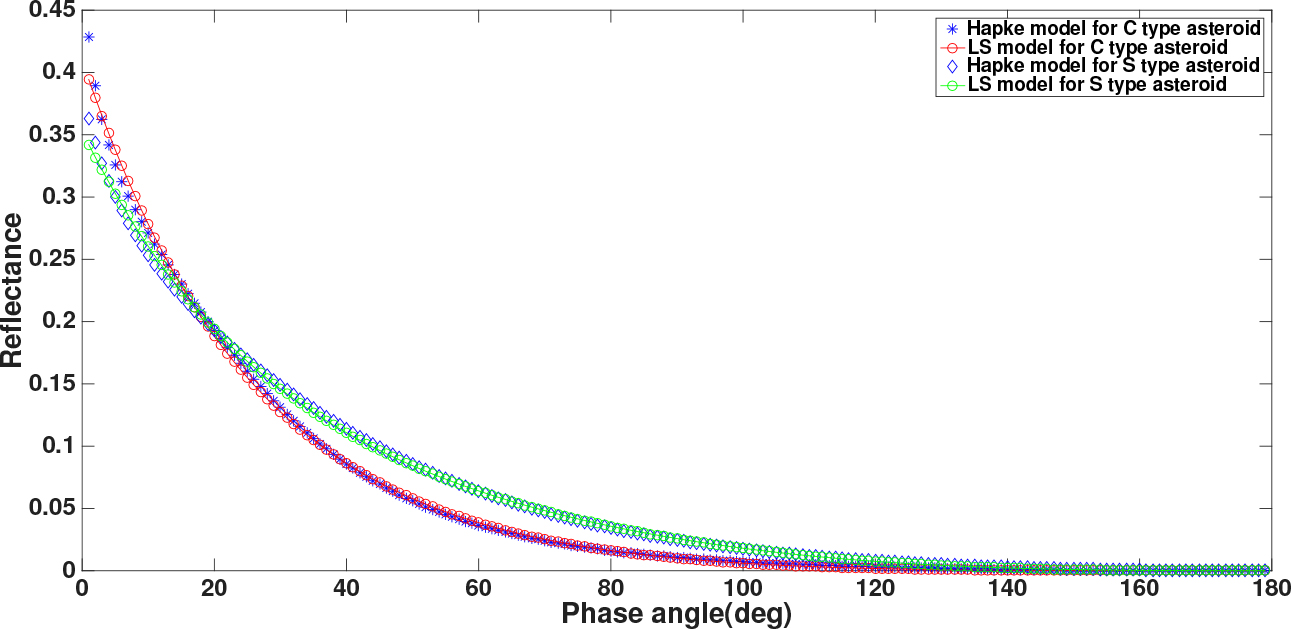Fig. 4 Phase curves for both C and S type asteroids derived from the Hapke and LS models.

In order to find taxonomic relations in the LS model for the two types of asteroids, we build test sets containing 1200 sample points for each type, which are selected respectively from the C and S type asteroids with the five corresponding Hapke parameters. Subsequently, equivalent parameters of the LS model for two types of asteroids are derived.

Then the PCA technique (Abdi & Williams ) is applied to determine the correlation of the four variables in the LS model and the results are presented in Table 4. The cumulative probability of previous variables of Z 1 and Z 2 is more than 95% and their dependence relations on the four variables of the LS model are formulated in Equation (7) and Equation (8), respectively. Eventually, the distributions of the two principal components for C and S type asteroids are shown in Figure 5 with a clear clustered feature. The regression lines for C and S type asteroids are shown in the same figure and their formulas are given in Equation (9) and Equation (10), respectively.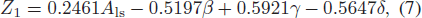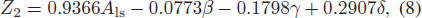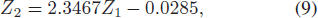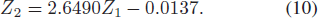Cluster distribution map of C (blue ‘∗’) and S (red ‘∗’) type asteroids. The regression line of C type is represented as a green line and the one of S type is shown as a pink line.

PCA Results

 Principal Component Z 1 Z 2 Z 3 Z 4 Percent Variance (%) 70.74 25.24 3.59 0.43 Cumulative Percent (%) 70.74 95.98 99.57 100

The PCA results show that the parameters of the LS model for C and S type asteroids appear clustered, which can be applied in asteroid taxonomy. Following this, we try to find an efficient way to classify the C and S type asteroids based on the LS model parameters in the subsequent subsection.

3.3. Identifying C and S types Based on the LS Model

As previously described, the principal components of four parameters in the LS model show clear clustering. Here we present an efficient technique to identify the taxonomy of an asteroid from its LS parameters. The LASSO method (Tibshirani ; Mei & Ling ; Musoro et al. ) in machine learning is commonly applied to search the best-fit solution with sparse non-zero variables.

Asteroid taxonomy from the LS parameters can be expressed in the LASSO format as a linear system of equations,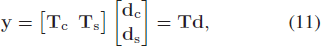where the coefficient matrix T = [T c, T s] consists of known LS parameters for both C and S type asteroids. In the context of machine learning, the coefficient matrix T is the training set. For any given LS parameters y, by solving the linear system (11) the vector d = [d c, d s] T will indicate the possible asteroid type.

As the LASSO method minimizes the following optimization problem with the l 1 regularization term,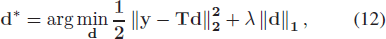a probability distribution can be derived to confirm that the asteroid with this group of LS parameters can be classified as C or S type. As a result of the employed l 1 regularization, which encourages small components of d to become exactly zero, the derived result d * presents sparse solutions with several non zero components, indicating the asteroid type.

First, the training set including a total of 2400 sample points is built by merging the LS model parameters for known C and S type asteroids with the respective 1200 sample points, where the LS model parameters are calculated equivalently from Hapke model parameters for known C and S type asteroids. Then we also generate a test set containing 200 points for known C and S type asteroids. Finally, by applying the gradient projection method (Figueiredo et al. ) to solve the problem in Equation (12), the taxonomy for the test set is derived.

As shown in Figures 6 and 7, the LASSO method can successfully derive the asteroid type. However, there are also some exceptional situations as shown in Figure 8. The probabilities for both C and S type are too close to discriminate them successfully. In all, there are 9 and 13 indeterminate cases for C and S type asteroids, respectively. Compared to the total test set with 200 sample points, the LASSO technique can identify C and S type asteroids from LS model parameters with an approximate probability of 90%. Furthermore, as shown in Figures 6 and 7, this method can also identify the similarity of two asteroids based on their LS model parameters.

Successfully derived indicators for C type asteroids by the LASSO method.

Successfully derived indicators for S type asteroids by the LASSO method.

Indeterminate indicators for C and S type asteroids by the LASSO method.

## 4. Conclusions

In this article, we analyze the two scattering models, Hapke and LS. Considering that the Hapke model is deduced with physical meanings and the LS model is efficient in numerical calculation, we numerically compare the two scattering models and confirm that the LS model can fit the Hapke model well in terms of phase curves. Additionally, for the shape determination of asteroids, the LS model can generate similar lightcurves with the same morphology as the Hapke model. Moreover, we primarily investigate two types asteroids, C and S type, classified based on the parameters of the Hapke model. By calculating the equivalent parameters of the LS model, we apply the PCA technique to show clear clustered features of two principal components of the LS model for C and S type asteroids. Moreover, we also introduce the method based on LASSO to classify an asteroid from LS scattering parameters to C or S type. This is very useful in real application. The shape, pole, rotational period, as well as the four LS scattering parameters can be derived from photometric data by an inversion process such as the Kaasalainen inversion method or the Cellinoid inversion process. Then by applying the technique presented in this article, an asteroid can be classified as C or S type in a fast way.

As now we only test C and S type asteroids, we want to explore more general taxonomy of asteroids and try to apply our technique to classify them in the future. We also expect that a new taxonomy method based on the LS model can be developed in the future.

 Abdi H. Williams L. J. 2010 Wiley Interdisciplinary Reviews: Computational Statistics 2 433 Besse S. Sunshine J. Staid M. et al. 2013 Icarus 222 229 Buratti B. Veverka J. 1983 Icarus 55 93 Cellino A. Muinonen K. Hestroffer D. Carbognani A. 2015 Planet. Space Sci. 118 221 DeMeo F. E. Carry B. 2014 Nature 505 629 Figueiredo M. A. T. Nowak R. D. Wright S. J. 2007 IEEE Journal of Selected Topics in Signal Processing 1 586 Hapke B. 1981 J. Geophys. Res. 86 3039 Hapke B. 1984 Icarus 59 41 Hapke B. 1986 Icarus 67 264 Hapke B. 2012 Theory of Reflectance and Emittance Spectroscopy Cambridge Cambridge Univ. Press Hapke B. Wells E. 1981 J. Geophys. Res. 86 3055 Helfenstein P. Veverka J. 1989 Asteroids II Binzel R. P. Gehrels T. Matthews M. S. 557 Karttunen H. 1989 A&A 208 314 Karttunen H. Bowell E. 1989 A&A 208 320 Li J. A’Hearn M. F. McFadden L. A. 2004 Icarus 172 415 Li J.-Y. Helfenstein P. Buratti B. Takir D. Clark B. E. 2015 Asteroid Photometry Michel P. DeMeo F. E. Bottke W. F. Asteroids IV University of Arizona Press Tucson 129 Li J.-Y. McFadden L. A. Parker J. W. et al. 2006 Icarus 182 143 Li J.-Y. A’Hearn M. F. Belton M. J. S. et al. 2007 Icarus 191 161 Li J.-Y. McFadden L. A. Thomas P. C. et al. 2010 Icarus 208 238 Li J.-Y. Le Corre L. Schröder S. E. et al. 2013 Icarus 226 1252 Lu X.-P. Cellino A. Hestroffer D. Ip W.-H. 2016 Icarus 267 24 Lu X.-P. Ip W.-H. 2015 Planet. Space Sci. 108 31 Lu X.-P. Ip W.-H. Huang X.-J. Zhao H.-B. 2017 Planet. Space Sci. 135 74 Lu X.-P. Zhao H.-B. You Z. 2013 RAA (Research in Astronomy and Astrophysics) 13 471 Lu X. Zhao H. You Z. 2014 Earth Moon and Planets 112 73 Mei X. Ling H.-B. 2009 IEEE 12th International Conference on Computer Vision 1436 Minnaert M. 1941 ApJ 93 403 Muinonen K. Lumme K. 2015 A&A 584 A23 Muinonen K. Penttilä A. Cellino A. et al. 2009 Meteoritics and Planetary Science 44 1937 Muinonen K. Wilkman O. Cellino A. Wang X. Wang Y. 2015 Planet. Space Sci. 118 227 Musoro J. Z. Zwinderman A. H. Puhan M. A. ter Riet G. Geskus R. B. 2014 BMC medical research methodology 14 116 Seeliger H. 1884 Astronomische Nachrichten 109 305 Takir D. Clark B. E. Drouet d’Aubigny C. et al. 2015 Icarus 252 393 Tibshirani R. 1996 Journal of the Royal Statistical Society B Methodological 58 267 Wang A. Wang X.-B. Muinonen K. Han X. L. Wang Y.-B. 2016 RAA (Research in Astronomy and Astrophysics) 16 180
Cite this article: Huang Xiang-Jie, Lu Xiao-Ping, Li Jian-Yang, Mei Bao, Hsia Chih-Hao, Zhao Hai-Bin. Scattering Law Analysis Based on Hapke and Lommel-Seeliger Models for Asteroidal Taxonomy. Cancer Biol Med. 2017; 10:106.

### Refbacks

• There are currently no refbacks.